# Arrow

)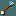Name arrow
Appearance arrow
Damage vs. small 1d6
Damage vs. large 1d6
To-hit bonus +0
Weapon skill bow
Size one-handed
Base price 2 zm
(+10/positive
enchant)
Weight 1
Material iron

An arrow is a projectile that can be thrown but is best used with a bow.

Besides the standard arrow shown here, there are several other types:

It is easy to obtain a large supply of arrows by untrapping an arrow trap.

As with many projectiles, arrows may break and disappear when they hit a monster. See Projectile#Breakage for more information.

## Using arrows

Although not necessary to throw or fire arrows, the best use of arrows is with an appropriate launcher.

The character must wield a bow and then throw arrows for them to be most effective. This process can be streamlined by quivering arrows and then using the fire command, but this is simply a convenience and is not necessary. However, crossbow bolts will not be fired from a wielded bow—they must be used with a crossbow instead.

The range for an arrow is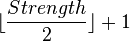$\lfloor \frac{Strength}{2} \rfloor +1$ when you are wielding a bow, and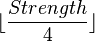$\lfloor \frac{Strength}{4} \rfloor$ when you are not:

 Strength: Range of arrow (with wielded bow) Range of arrow (without wielded bow) 3 4, 5 6, 7 8, 9 10, 11 12, 13 14, 15 16, 17 18–18/31 18/31–21 22, 23 24, 25 2 3 4 5 6 7 8 9 10 11 12 13 0 1 1 2 2 3 3 4 4 5 5 6

Along with other cursed missile weapons or launchers, cursed arrows occasionally "slip from your hand" when you throw or fire them, and end up on the ground.

Since arrows are used with a bow, they are affected by bow skill.

Bow
Max Role
Basic
Skilled
Expert

### Multishot

The number of arrows shot at once depends on the character's bow skill. You will shoot 1 arrow at a time at unskilled or basic skill, up to 2 arrows at skilled, and up to 3 at expert. There is a +1 bonus to this counter for rangers, and an additional +1 for elven characters shooting elven arrows from an elven bow, orcish characters shooting orcish arrows from an orcish bow, and samurai shooting ya from a yumi. This means, for example, an elven ranger using elven arrows and an elven bow can fire up to 5 arrows at once.

## Average damage calculation

The average damage calculations in the following table do not include bonuses from weapon skills or from using a blessed weapon against undead or demons. However, these bonuses apply per arrow, so they may cause far more damage than when attacking in melee.

The calculations for poisoned arrows assume the monster is not poison resistant.

Weapon Number of arrows fired at once
1 1d2 (average 1.5) 1d3 (average 2) 1d4 (average 2.5)
+0 arrows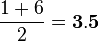$\frac{1+6}{2}=\bold{3.5}$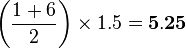$\left (\frac{1+6}{2}\right )\times 1.5=\bold{5.25}$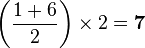$\left (\frac{1+6}{2}\right )\times 2=\bold{7}$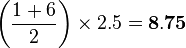$\left (\frac{1+6}{2}\right )\times 2.5=\bold{8.75}$
+7 arrows$\frac{1+6}{2}+7=\bold{10.5}$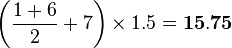$\left (\frac{1+6}{2}+7\right )\times 1.5=\bold{15.75}$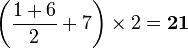$\left (\frac{1+6}{2}+7\right )\times 2=\bold{21}$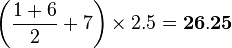$\left (\frac{1+6}{2}+7\right )\times 2.5=\bold{26.25}$
poisoned +0 arrows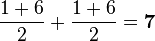$\frac{1+6}{2}+\frac{1+6}{2}=\bold{7}$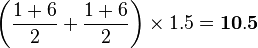$\left (\frac{1+6}{2}+\frac{1+6}{2}\right )\times 1.5=\bold{10.5}$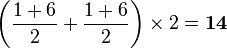$\left (\frac{1+6}{2}+\frac{1+6}{2}\right )\times 2=\bold{14}$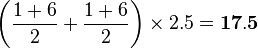$\left (\frac{1+6}{2}+\frac{1+6}{2}\right )\times 2.5=\bold{17.5}$
poisoned +7 arrows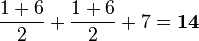$\frac{1+6}{2}+\frac{1+6}{2}+7=\bold{14}$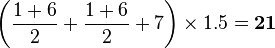$\left (\frac{1+6}{2}+\frac{1+6}{2}+7\right )\times 1.5=\bold{21}$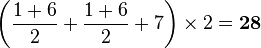$\left (\frac{1+6}{2}+\frac{1+6}{2}+7\right )\times 2=\bold{28}$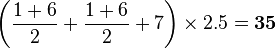$\left (\frac{1+6}{2}+\frac{1+6}{2}+7\right )\times 2.5=\bold{35}$

## Encyclopedia entry

I shot an arrow into the air,
It fell to earth, I knew not where;
For, so swiftly it flew, the sight
Could not follow it in its flight.

I breathed a song into the air,
It fell to earth, I knew not where;
For who has sight so keen and strong
That it can follow the flight of song?

Long, long afterward, in an oak
I found the arrow still unbroke;
And the song, from beginning to end,
I found again in the heart of a friend.

[ The Arrow and the Song,